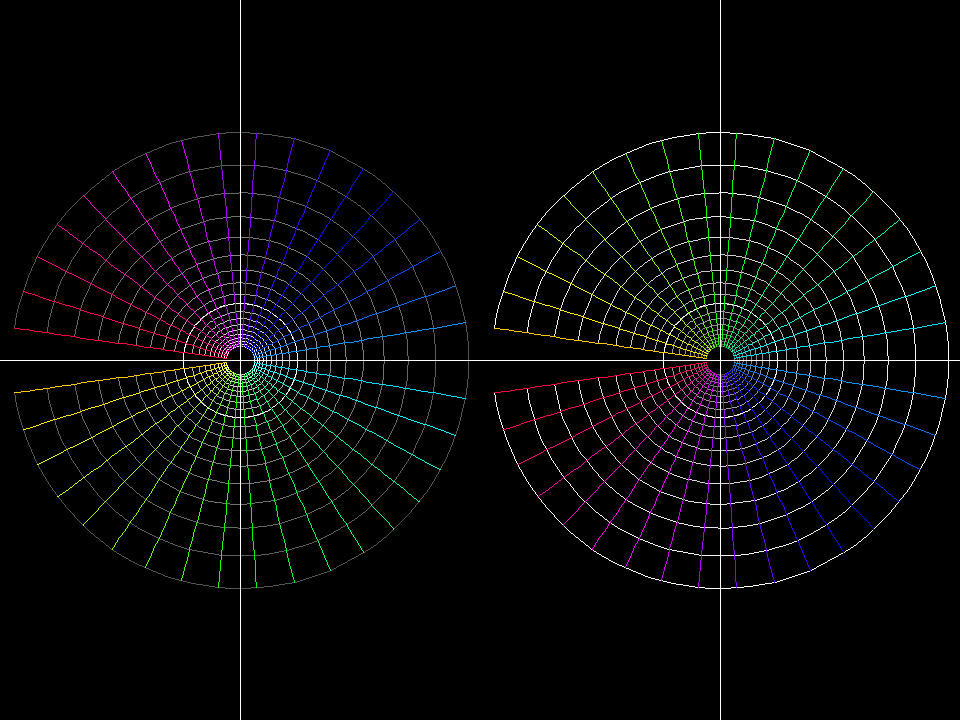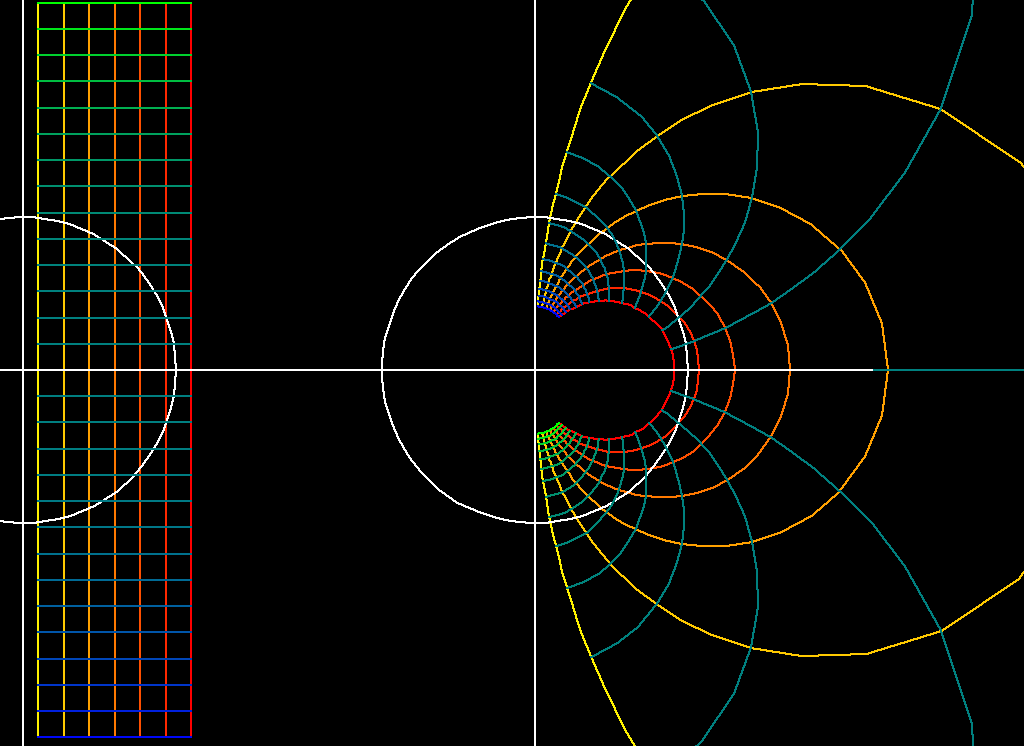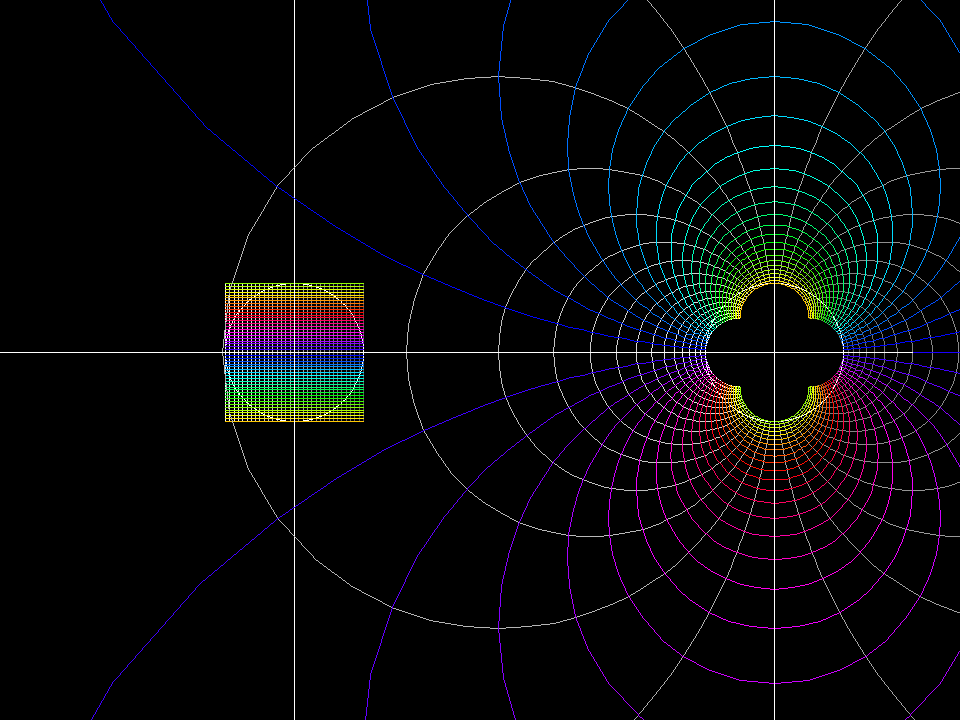# Complex Inversion

Complex inversion is the function `1/z`.

## Complex Inversion on Polar GridComplex inversion on polar grid. Left is pre-image. Right is the image. Note that radial lines mappeds to the opposite side over origin, and small circles near origin becomes big outter circles. Complex inversion essentially “swaps” the plane inside-out and rotate it 180 degrees around origin.
• polar grid, `{r, 1/4 + 3 a/4 , 4}`, `{φ, -3 + a*3 , 3}`
• Morph parameter: `{a,0,1}`Complex inversion on polar grid projected to Riemann sphere. When visualized on Riemann sphere, points on bottom of the sphere goes to the top, and vice versa, and a 180 degree rotation is done on the vertical axes of the sphere.

## Complex Inversion on Rectangle GridPlot of 1/z. domain: cartesian square grid, 0.1 + c ≦ Re(z) ≦ 1.1 + c, -2 ≦ Im(z) ≦ 2 range : parameterlines are circles in the Gaussian plane. morph : 0 ≦ c ≦ 0.5

Observe that all parameter circles are tangential to the coordinate axes at 0. Since we use the inverse map to visualize what happens near infinity, we translate this tangential property of the circles back into the domain and say: “parallel lines are tangent to each other at infinity”.

• function: `z → 1/z`
• domain: cartesian square grid, `0.1 + c ≦ Re(z) ≦ 1.1 + c`, `-2 ≦ Im(z) ≦ 2`
• range : image is shown on the Riemann sphere.
• morph : `0 ≦ c ≦ 0.5`

The inverse function maps any straight line in the domain to a circle through 0. Since we show domain and range we cannot use full lines. The stereo image shows very well that all the (partial) circles lie on the unit sphere and the full circles would go through the south pole (the stereographic image of 0).map: z ⟶ f(z) = 1/z domain: cartesian grid with -1 < re(z) < 1, -1-a*2 < im(z) < +1+a*2 morph: 0 < a < 1 range: Gaussian plane Note that all parameter lines are mapped to circles (in the range), which are tangent to the coordinate axes. The outside of the parameter rectangle is mapped to the black domain inside the unit circle (in the range). The image of the parameter rectangle is the outside of this black domain, including infinity.map: z ⟶ f(z) = 1/z domain: cartesian grid with -1 < re(z) < 1, -1-a*2 < im(z) < +1+a*2 morph: 0 < a < 1 range: Riemann Sphere, anaglyph.

One can see on the Riemann sphere that infinity is covered without any singular behavior. This is an example of the fact that all rational functions are better visualized on the Riemann sphere than in the Gaussian plane.

It is an important property of the inverse function that it maps lines and circles in the Gaussian plane to circles on the Riemann sphere. This is no surprise since the inverse function as a map from the Riemann sphere to the Riemann sphere is just a 180 degree rotation. For computations use this representation of a circle: { z | (z-m)*conjugate(z-m) = r^2 }.

z_inversion.pdf